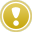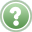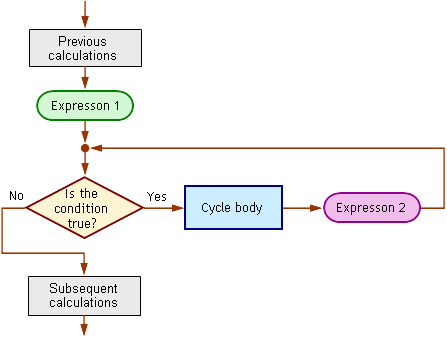English

MQL4 BookOperatorsCycle Operator 'for'

# Cycle Operator 'for'

Another cycle operator is the operator 'for'.

## Format of the Operator 'for'

The full-format cycle operator 'for' consists of the header that contains Expression_1, Condition and Expression_2, and of the executable cycle body enclosed in braces.

`  for (Expression_1; Condition; Expression_2)         // Cycle operator header      {                                            // Opening brace      Block of operators                           // Cycle body may consist ..      composing the cycle body                     //.. of several operators      }                                            // Closing brace`

If the cycle body in the operator 'for' consists of only one operator, braces can be omitted.

`  for (Expression_1; Condition; Expression_2)         // Cycle operator header      One operator, cycle body                     // Cycle body is one operator`

Expression_1, Condition and/or Expression_2 can be absent. In any case, the separating character ";" (semicolon) must remain in the code.

`  for ( ; Condition; Expression_2)                    // No Expression_1      {                                            // Opening brace      Block of operators                           // Cycle body may consist ..      composing the cycle body                     //.. of several operators      }                                            // Closing brace// - ---------------------------------------------------------------------------------  for (Expression_1; ; Expression_2)                 // No Condition      {                                            // Opening brace      Block of operators                           // Cycle body may consist ..      composing the cycle body                     //.. of several operators      }                                            // Closing brace// - ---------------------------------------------------------------------------------  for ( ; ; )                                          // No Expressions or Condition      {                                            // Opening brace      Block of operators                           // Cycle body may consist ..      composing the cycle body                     //.. of several operators      }                                            // Closing brace`

## Execution Rule of the Operator 'for'As soon as the control is passed to the operator 'for', the program executes Expression_1. As long as the Condition of the operator 'for' is true: pass the control to the first operator in the cycle body; as soon as all operators in the cycle body are executed, the program must execute Expression_2 and pass the control to the header for testing the Condition for being true. If the Condition of the operator 'for' is false, then: the program must pass the control to the operator that follows the operator 'for'.

Let's consider how the cycle operator 'for' works. For this, let's solve a problem.Problem 13. We have a sequence of integers: 1 2 3 4 5 6 7 8 9 10 11 ... Compose a program that would calculate the sum of the elements of this sequence starting from N1 and finishing at N2.

This problem is easy to solve in terms of mathematics. Suppose we want to calculate the sum of elements from the third through the seventh one. The solution will be: 3 + 4 + 5 + 6 + 7 = 25. However, this solution is good only for a particular case, when the numbers of the first and the last elements composing the sum are equal to 3 and 7, respectively. A program solving this problem must be composed in such a way that, if we want to calculate the sum within another interval of the sequence (for example, from the 15th to 23rd element), we could easily replace the numeric values of the elements in a location in the program without modifying program lines in other locations. Below is one of the versions of such a program (script sumtotal.mq4):

`//-----------------------------------------------------------------------------// sumtotal.mq4// The code should be used for educational purpose only.//-----------------------------------------------------------------------------int start()                          // Special function start()  {//-----------------------------------------------------------------------------   int   Nom_1,                               // Number of the first element   Nom_2,                               // Number of the second element   Sum,                                 // Sum of the numbers   i;                                   // Formal parameter (counter)//-----------------------------------------------------------------------------   Nom_1=3;                              // Specify numeric value   Nom_2=7;                              // Specify numeric value   for(i=Nom_1; i<=Nom_2; i++)           // Cycle operator header     {                                   // Brace opening the cycle body      Sum=Sum + i;                       // Sum is accumulated      Alert("i=",i,"  Sum=",Sum);        // Display on the screen     }                                   // Brace closing the cycle body//------------------------------------------------------------------------------   Alert("After exiting the cycle, i=",i,"  Sum=",Sum);// Display on the screen   return;                               // Exit start()  }//------------------------------------------------------------------------------`

In the first lines of the program, variables are declared (the comments explain the sense of each variable). In the lines

`   Nom_1 = 3;                           // Specify numeric value   Nom_2 = 7;                           // Specify numeric value`

the numeric values of the first and of the last element of the range are defined. Please note that these values aren't specified anywhere else in the program. If necessary, you can easily change these values (in one location) without changing the code in other lines, so that the problem can be solved for another range. After the last of these operators has been executed, the control is passed to the cycle operator 'for':

`   for(i=Nom_1; i<=Nom_2; i++)          // Cycle operator header     {                                  // Brace opening the cycle body      Sum = Sum + i;                    // Sum is accumulated      Alert("i=",i,"  Sum=",Sum);       // Display on the screen     }                                  // Brace closing the cycle body`

The key phrase - the execution rule of the operator 'for' - is as follows: "Starting from .., as long as .., step .., perform the following: ..". The use of 'step..' is applicable, if we use a storage counter, for example, i++ или i=i+2, as Expression_2. In our example, the key phrase is as follows: Starting from i that is equal to Nom_1, as long as i is less or equal to Nom_2, step 1, perform the following: (and then - the analysis of the cycle body).

According to the execution rule of the operator 'for' the following will be executed in this block of the program:

1. Execution of Expression_1:

`   i=Nom_1`

Variable i will take the numeric value of variable Nom_1, i.e., integer 3. Expression_1 is executed only once - when the control is passed to the operator 'for'. Expression_1 is not involved in any subsequent events.

2. The Condition is tested:

`   i<=Nom_2`

The value of variable Nom_2 at the first iteration is integer 7, whereas the value of variable i is equal to 3. This means that the Condition is true (since 3 is less than 7), i.e., the control will be passed to the cycle body to execute it.

3. In our example, the cycle body consists of two operators that will be executed one by one:

`   Sum = Sum + i;                    // Sum is accumulated   Alert("i=",i,"  Sum=",Sum);       // Display on the screen`

Variable Sum, at its initialization, did not take any initial value, so its value is equal to zero before the first iteration starts. During calculations, the value of variable Sum is increased by the value of i, so it is equal to 3 at the end of the first iteration. You can see this value in the box of the function Alert():

 i=3 Sum=3

4. The last event that happens during execution of the cycle operator is the execution of Expression_2:

`   i++`

The value of variable i is increased by one. This is the end of the first iteration, the control is passed to test the Condition.

Then the second iteration starts, steps 2-4. During calculations, the variables take new values. Particularly, at the second iteration, the value of variable i is equal to 4, whereas Sum is equal to 7. The corresponding message will appear, too. Generally, the program will develop according to the block diagram below:Fig. 43. Block diagram of the operator-'for' execution in program sumtotal.mq4.

The program will cyclically repeat the execution of the cycle operator, until the Condition becomes false. This will happen, as soon as the value of variable i is equal to 8, i.e., exceeds the preset value 7 of variable Nom_2. In this case, the control will be passed outside the operator 'for', namely, to the line:

`   Alert("After exiting the cycle, i=",i,"  Sum=",Sum);// Display on the screen`

and further, to execution of the operator 'return' that ends the operation of the special function start().

You can use this program to calculate the sum of any other range of values. For example, if you replace the constants 3 and 7 with 10 and 15, respectively, the execution of the program will result in calculation of the sum of values within this range.

In some programming languages, the variables specified in the cycle operator header lose their values after exiting the cycle. This is not the case in MQL4. All variables that are involved in any calculations are valid and keep their values after exiting the cycle. At the same time, there are some particularities concerning the values of variables that are involved in calculations.

Please note the last two messaging lines that remain after all calculations are complete and the script is unloaded:

 i=7 Sum=25
 After exiting the cycle, i=8 Sum=25

The former of the above two messages had appeared at the stage of the last iteration, before the cycle was complete, whereas the latter of them had been given by the last function Alert() before the program was terminated. It is remarkable that these lines display different values of variable i. During the cycle execution, this value is equal to 7, but it is equal to 8 after exiting the cycle. In order to understand, why it happens this way, let's refer to Fig. 43 once again.

The last (in this case, the fifth) iteration starts with testing the Condition. The value of i is equal to 7 at this moment. Since 7 does not exceed the value of variable Nom_2, the Condition is true and the cycle body will be executed. As soon as operators composing the cycle body are executed, the last operation is performed: Expression_2 is executed. This must be emphasized once again:The last event taking place at each iteration of the operator-'for' execution is the calculation of Expression_2.

This results in increasing of the value of variable i by 1, so that this value becomes equal to 8. The subsequent test of the Condition (at the beginning of the next iteration) confirms that the Condition is false now. This is why the control will be passed outside the cycle operator after testing. At the same time, variable i exits the cycle operator with the value 8, whereas the value of variable Sum remain to be equal to 25, because the cycle body is not executed at this stage. This must be considered in situations where the amount of iteration is not known in advance, so that the programmer is going to estimate it by the value of the variable calculated in Expression_2.

We can consider a situation that produces looping to be an exceptional case. This becomes possible at various errors. For example, if you use the following operator as Expression_2 by error:

`   i--`

the value of the counter at each iteration will decrease, so that the Condition will never become false.

Another error can be an incorrectly composed Condition:

`for(i=Nom_1; i>=Nom_1; i++)              // Cycle operator header`

In this case, the value of variable i will always exceed or be equal to the value of variable Nom_1, so that the Condition is always true.

## Interchangeability of Cycle Operators 'while' and 'for'

The use of one operator or another fully depends on the decision made by the programmer. The only thing we will note is that these operators are interchangeable; it is often necessary just to give another brush to the code of your program.

For example, we used the cycle operator 'for' to solve Problem 13:

`   for (i=Nom_1; i<=Nom_2; i++)         // Cycle operator header     {                                  // Brace opening the cycle body      Sum = Sum + i;                    // Sum is accumulated      Alert("i=",i,"  Sum=",Sum);       // Display on the screen     }                                  // Brace closing the cycle body`

Below is an example how the same fragment of the code may look if we use the operator 'while':

`   i=Nom_1;                             // Оператор присваивания   while (i<=Nom_2)                     // Cycle operator header     {                                  // Brace opening the cycle body      Sum = Sum + i;                    // Sum is accumulated      Alert("i=",i,"  Sum=",Sum);       // Display on the screen      i++;                              // Increment of the element number     }                                  // Brace closing the cycle body`

As is easy to see, it's sufficient just to move Expression_1 into the line that precedes the cycle operator, whereas Expression_2 should be specified as the last in the cycle body. You can change the exemplary program yourself and launch it for execution, in order to see that the results are the same for both versions.Cycle Operator 'while'Operator 'break'